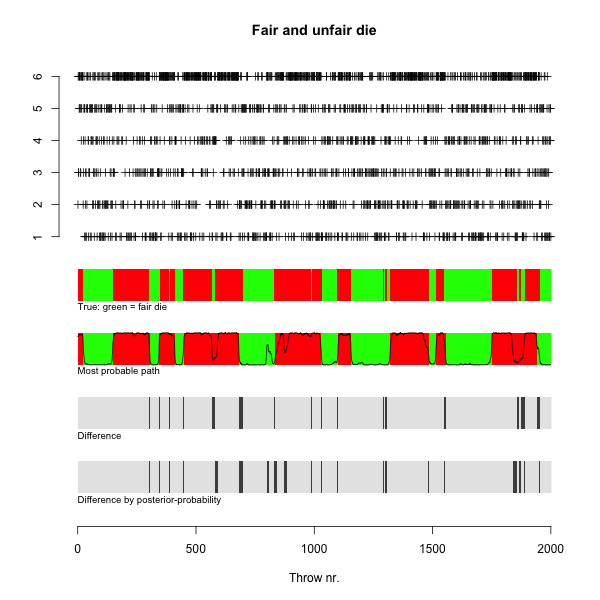# Hidden Markov Models - the Unfair Casino¶

## Model¶

1. State Space (suppose we have two: fair dice /loaded dice)
2. Switch between two states is according to a given matrix (Markov transition matrix)
3. Output is probabilistic but depends on the state (fair/loaded)
4. Want to guess the hidden state (fair/loaded) from the output observed (here tosses of the die).

## Examples in R¶

>  require(HMM)
>  nSim = 2000
>  States = c("Fair", "Unfair")
>  Symbols = 1:6
>  transProbs = matrix(c(0.99, 0.01, 0.02, 0.98), c(length(States),
+      length(States)), byrow = TRUE)
>  emissionProbs = matrix(c(rep(1/6, 6), c(rep(0.1, 5), 0.5)),
+      c(length(States), length(Symbols)), byrow = TRUE)
>  hmm = initHMM(States, Symbols, transProbs = transProbs, emissionProbs = em
issionProbs)
>  sim = simHMM(hmm, nSim)
>  vit = viterbi(hmm, sim$observation) > f = forward(hmm, sim$observation)
>  b = backward(hmm, sim$observation) > i <- f[1, nSim] > j <- f[2, nSim] > probObservations = (i + log(1 + exp(j - i))) > posterior = exp((f + b) - probObservations) > x = list(hmm = hmm, sim = sim, vit = vit, posterior = posterior) > mn = "Fair and unfair die" > xlb = "Throw nr." > ylb = "" >  ### R code for cut and paste¶ rm(X) #############you must install the package HMM #### dishonestCasino() require(HMM) nSim = 2000 States = c("Fair", "Unfair") Symbols = 1:6 transProbs = matrix(c(0.99, 0.01, 0.02, 0.98), c(length(States), length(States)), byrow = TRUE) emissionProbs = matrix(c(rep(1/6, 6), c(rep(0.1, 5), 0.5)), c(length(States), length(Symbols)), byrow = TRUE) hmm = initHMM(States, Symbols, transProbs = transProbs, emissionProbs = emissionProbs) sim = simHMM(hmm, nSim) vit = viterbi(hmm, sim$observation)
f = forward(hmm, sim$observation) b = backward(hmm, sim$observation)
i <- f[1, nSim]
j <- f[2, nSim]
probObservations = (i + log(1 + exp(j - i)))
posterior = exp((f + b) - probObservations)
x = list(hmm = hmm, sim = sim, vit = vit, posterior = posterior)
##Plotting simulated throws at top
mn = "Fair and unfair die"
xlb = "Throw nr."
ylb = ""


>  plot(x$sim$observation, ylim = c(-7.5, 6), pch = 3, main = mn,
+      xlab = xlb, ylab = ylb, bty = "n", yaxt = "n")
>  axis(2, at = 1:6)
>   text(0, -1.2, adj = 0, cex = 0.8, col = "black", "True: green = fair die"
)
>   for (i in 1:nSim) {
+      if (x$sim$states[i] == "Fair")
+          rect(i, -1, i + 1, 0, col = "green", border = NA)
+      else rect(i, -1, i + 1, 0, col = "red", border = NA)
+     }
>  text(0, -3.2, adj = 0, cex = 0.8, col = "black", "Most probable path")
>  for (i in 1:nSim) {
+      if (x$vit[i] == "Fair") + rect(i, -3, i + 1, -2, col = "green", border = NA) + else rect(i, -3, i + 1, -2, col = "red", border = NA) + } > text(0, -5.2, adj = 0, cex = 0.8, col = "black", "Difference") > differing = !(x$sim$states == x$vit)
>  for (i in 1:nSim) {
+      if (differing[i])
+          rect(i, -5, i + 1, -4, col = rgb(0.3, 0.3, 0.3),
+              border = NA)
+      else rect(i, -5, i + 1, -4, col = rgb(0.9, 0.9, 0.9),
+          border = NA)
+         }
>   points(x$posterior[2, ] - 3, type = "l") > text(0, -7.2, adj = 0, cex = 0.8, col = "black", "Difference by posterior -probability") > differing = !(x$sim$states == x$vit)
>   for (i in 1:nSim) {
+      if (posterior[1, i] > 0.5) {
+          if (x$sim$states[i] == "Fair")
+              rect(i, -7, i + 1, -6, col = rgb(0.9, 0.9, 0.9),
+                border = NA)
+          else rect(i, -7, i + 1, -6, col = rgb(0.3, 0.3, 0.3),
+              border = NA)
+       }
+       else {
+           if (x$sim$states[i] == "Unfair")
+               rect(i, -7, i + 1, -6, col = rgb(0.9, 0.9, 0.9),
+                 border = NA)
+           else rect(i, -7, i + 1, -6, col = rgb(0.3, 0.3, 0.3),
+              border = NA)
+        }
+      }
>Source :

### R code for cut and paste¶

plot(x$sim$observation, ylim = c(-7.5, 6), pch = 3, main = mn,
xlab = xlb, ylab = ylb, bty = "n", yaxt = "n")
axis(2, at = 1:6)
#######Simulated, which die was used (truth)####################
text(0, -1.2, adj = 0, cex = 0.8, col = "black", "True: green = fair die")
for (i in 1:nSim) {
if (x$sim$states[i] == "Fair")
rect(i, -1, i + 1, 0, col = "green", border = NA)
else rect(i, -1, i + 1, 0, col = "red", border = NA)
}
########Most probable path (viterbi)#######################
text(0, -3.2, adj = 0, cex = 0.8, col = "black", "Most probable path")
for (i in 1:nSim) {
if (x$vit[i] == "Fair") rect(i, -3, i + 1, -2, col = "green", border = NA) else rect(i, -3, i + 1, -2, col = "red", border = NA) } ##################Differences: text(0, -5.2, adj = 0, cex = 0.8, col = "black", "Difference") differing = !(x$sim$states == x$vit)
for (i in 1:nSim) {
if (differing[i])
rect(i, -5, i + 1, -4, col = rgb(0.3, 0.3, 0.3),
border = NA)
else rect(i, -5, i + 1, -4, col = rgb(0.9, 0.9, 0.9),
border = NA)
}

#################Posterior-probability:#########################
points(x$posterior[2, ] - 3, type = "l") ###############Difference with classification by posterior-probability:############ text(0, -7.2, adj = 0, cex = 0.8, col = "black", "Difference by posterior-probability") differing = !(x$sim$states == x$vit)
for (i in 1:nSim) {
if (posterior[1, i] > 0.5) {
if (x$sim$states[i] == "Fair")
rect(i, -7, i + 1, -6, col = rgb(0.9, 0.9, 0.9),
border = NA)
else rect(i, -7, i + 1, -6, col = rgb(0.3, 0.3, 0.3),
border = NA)
}
else {
if (x$sim$states[i] == "Unfair")
rect(i, -7, i + 1, -6, col = rgb(0.9, 0.9, 0.9),
border = NA)
else rect(i, -7, i + 1, -6, col = rgb(0.3, 0.3, 0.3),
border = NA)
}
}